## John and Jivanti are playing with the marbles in  the playground. They together have 45 marbles  and John has 15 marbles more than Jivanti.

This question is exactly same as Example 1 (i) - Chapter 4 Class 10 - Quadratic Equations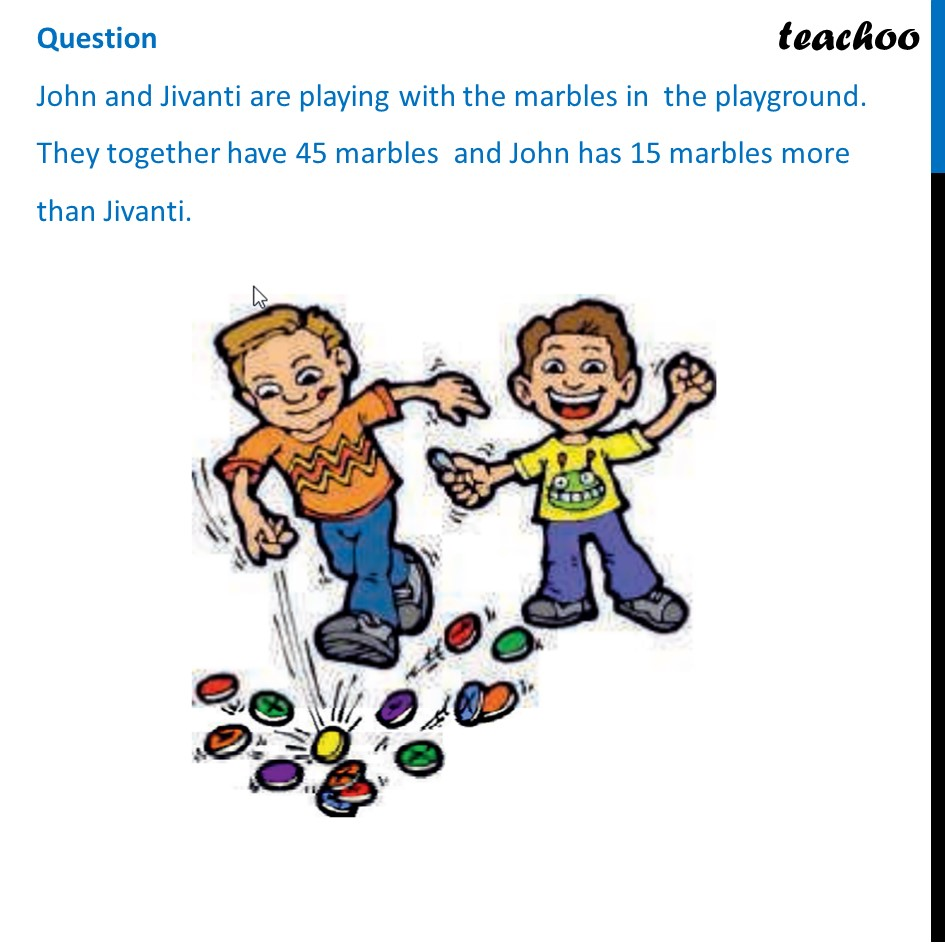## (d) 5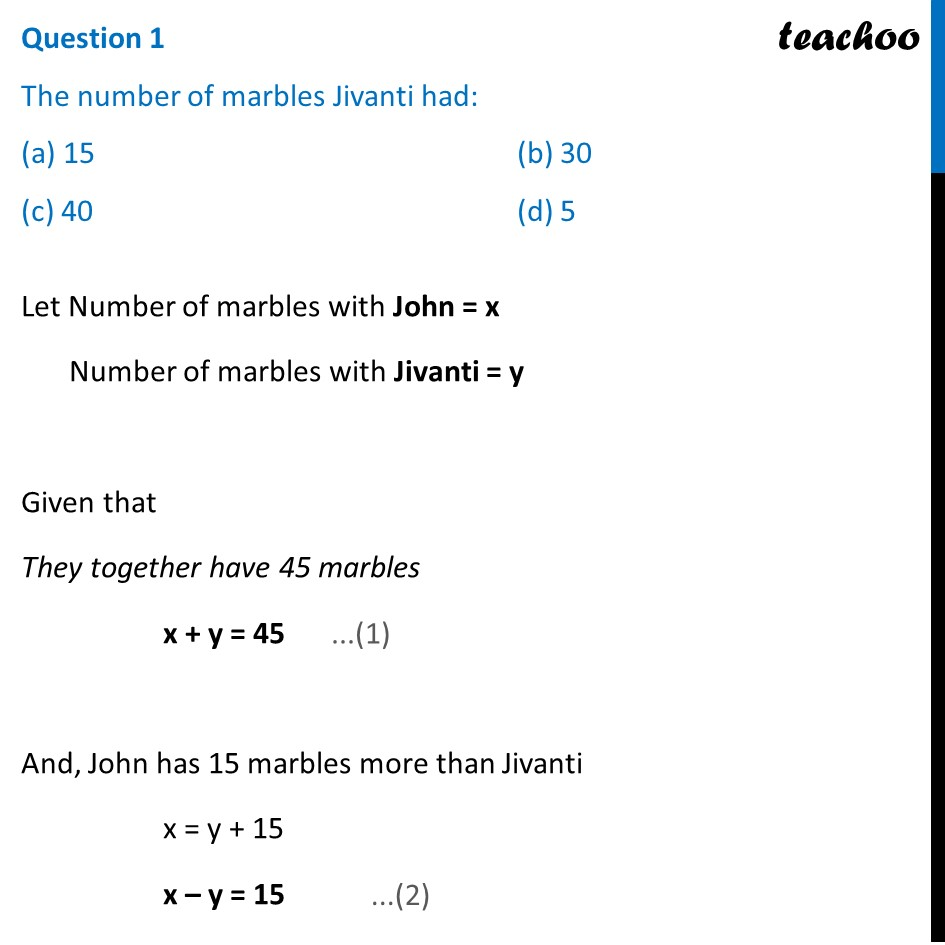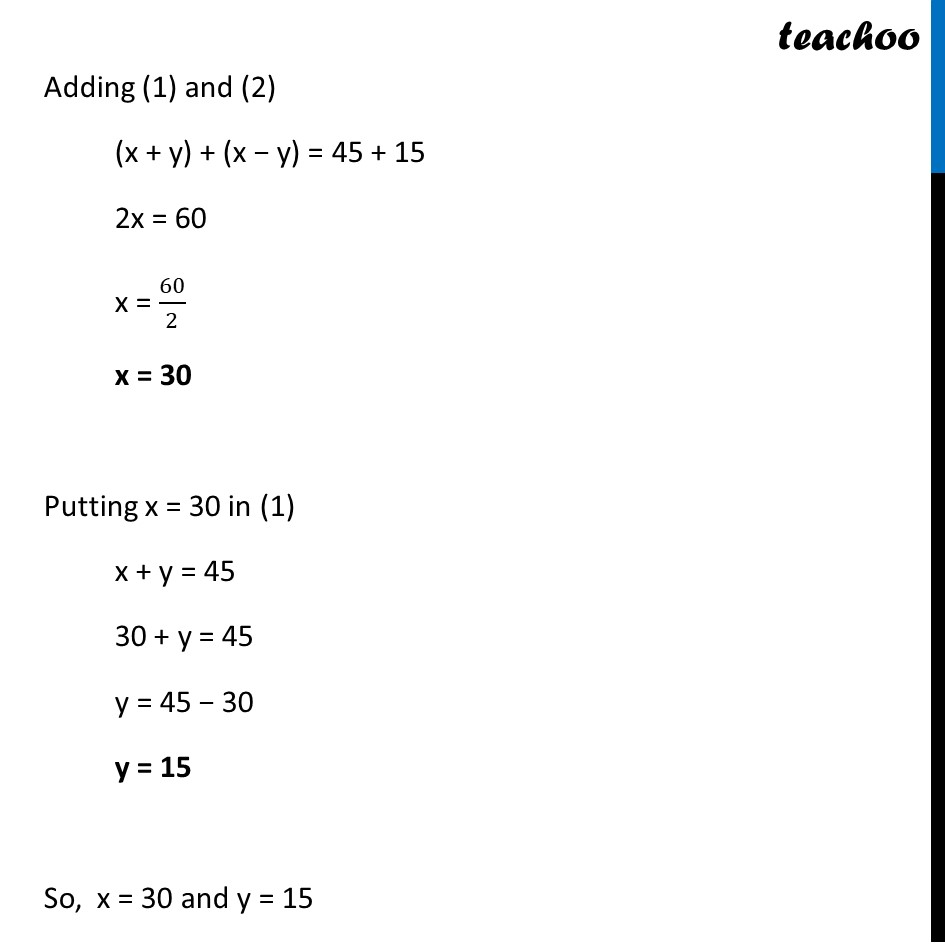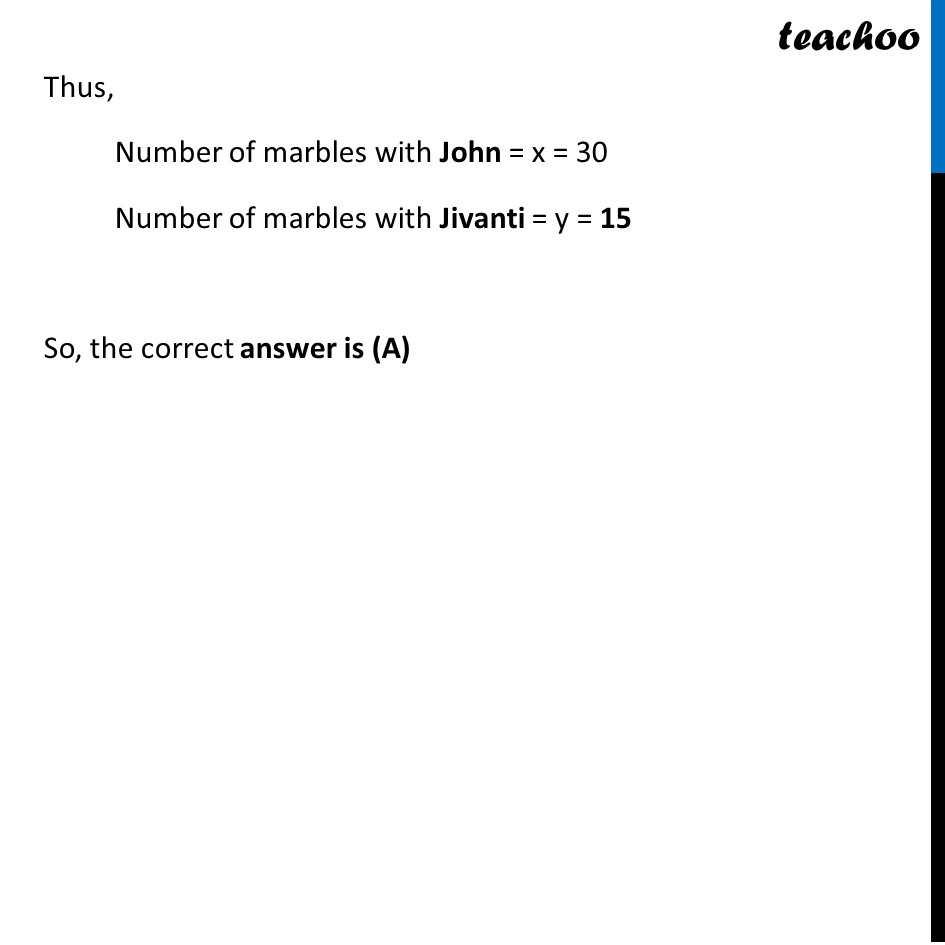## (d) 20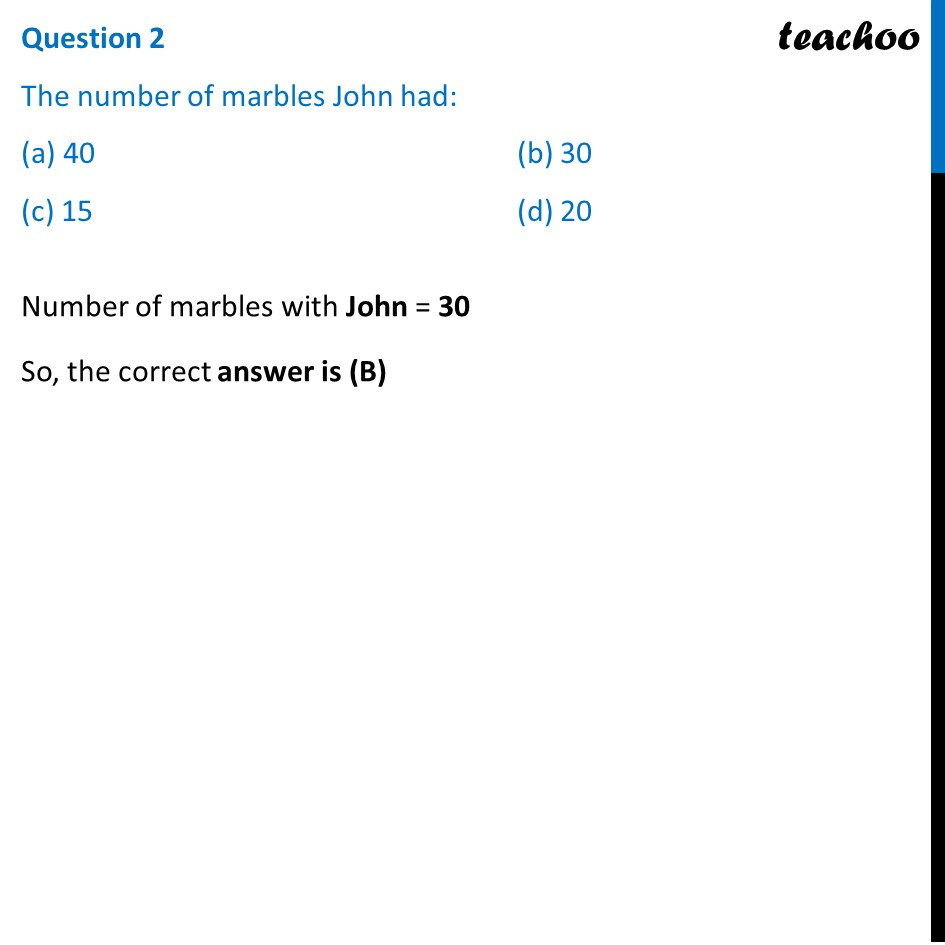## (d) 35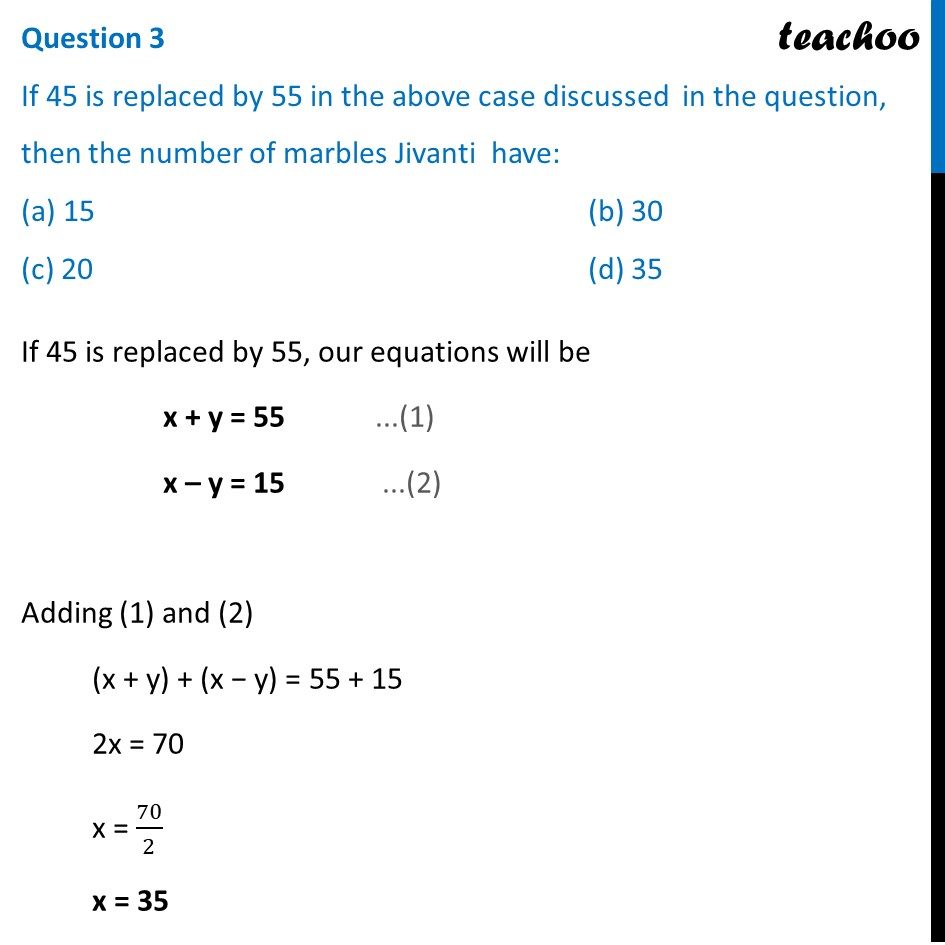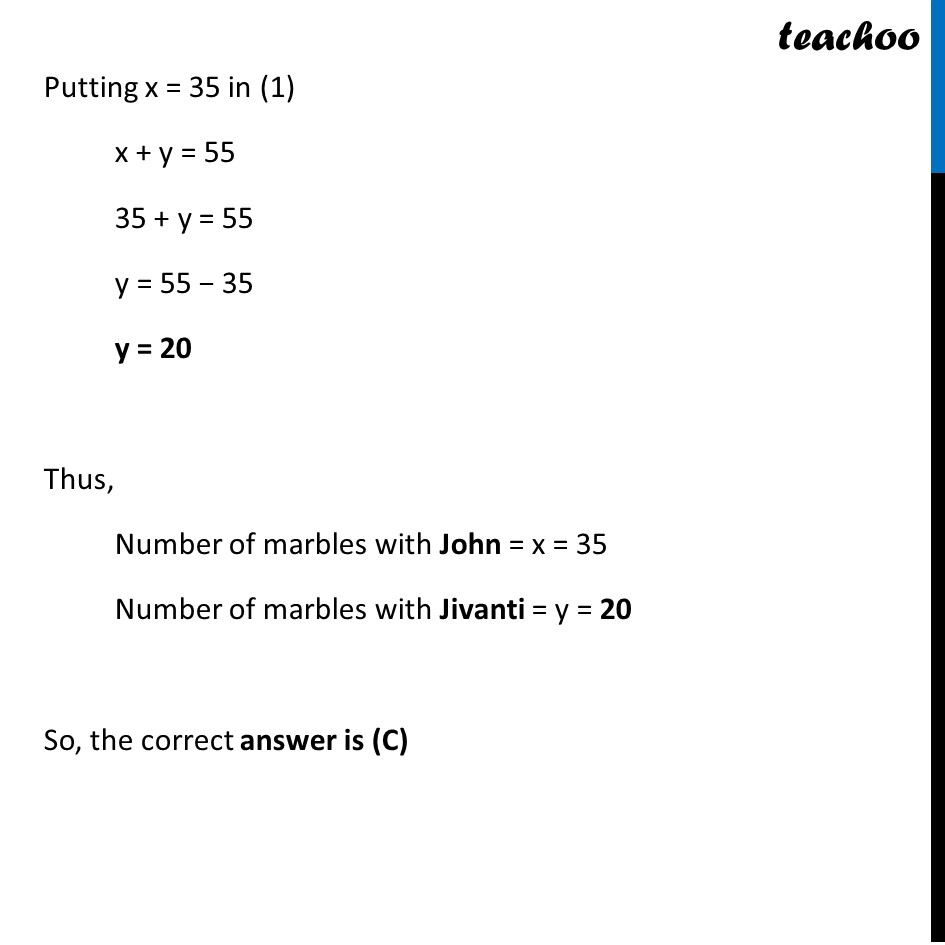## (d) 35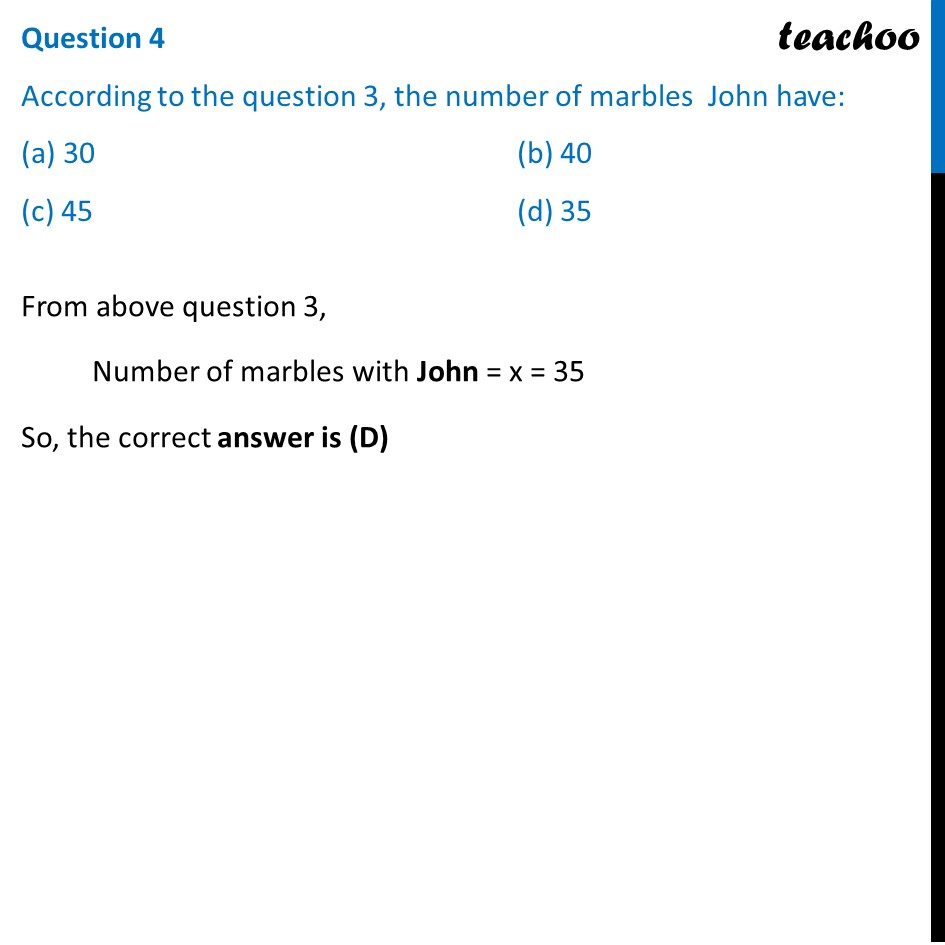## (d) None of the above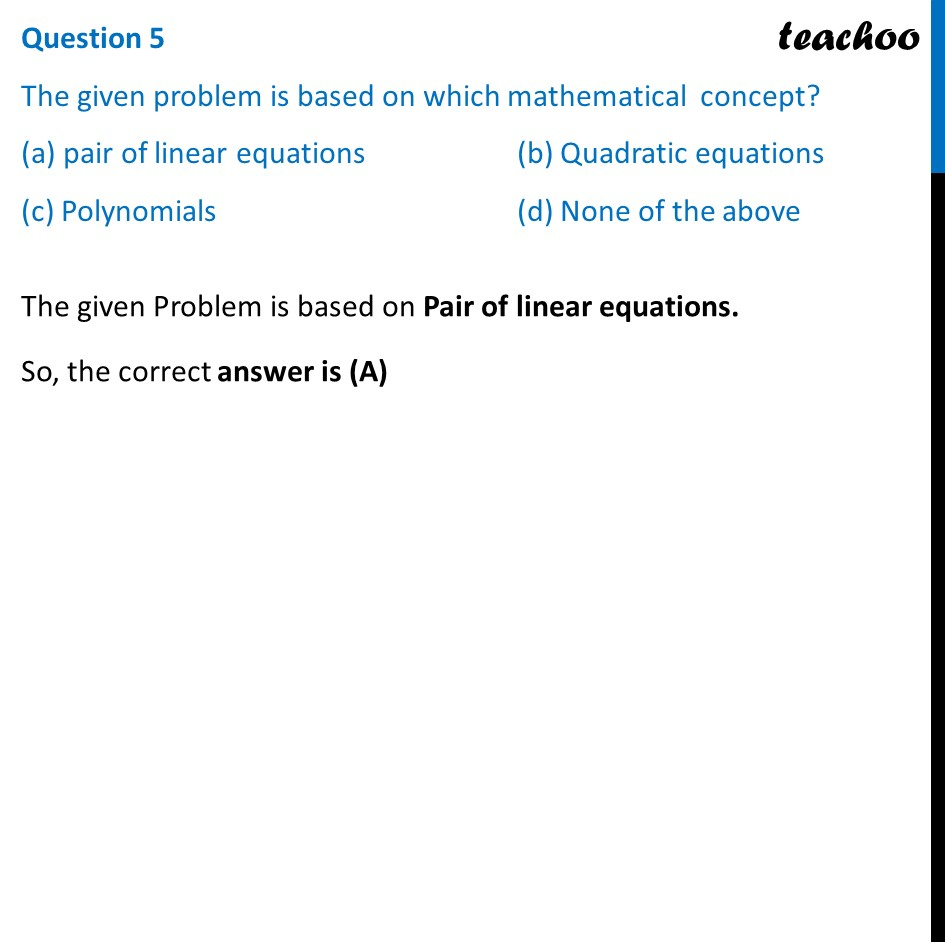1. Chapter 3 Class 10 Pair of Linear Equations in Two Variables (Term 1)
2. Serial order wise
3. Case Based Questions (MCQ)

Transcript

Question John and Jivanti are playing with the marbles in the playground. They together have 45 marbles and John has 15 marbles more than Jivanti. Question 1 The number of marbles Jivanti had: (a) 15 (b) 30 (c) 40 (d) 5 Let Number of marbles with John = x Number of marbles with Jivanti = y Given that They together have 45 marbles x + y = 45 And, John has 15 marbles more than Jivanti x = y + 15 x – y = 15 Adding (1) and (2) (x + y) + (x − y) = 45 + 15 2x = 60 x = 60/2 x = 30 Putting x = 30 in (1) x + y = 45 30 + y = 45 y = 45 − 30 y = 15 So, x = 30 and y = 15 Thus, Number of marbles with John = x = 30 Number of marbles with Jivanti = y = 15 So, the correct answer is (A) Question 2 The number of marbles John had: (a) 40 (b) 30 (c) 15 (d) 20 Number of marbles with John = 30 So, the correct answer is (B) Question 3 If 45 is replaced by 55 in the above case discussed in the question, then the number of marbles Jivanti have: (a) 15 (b) 30 (c) 20 (d) 35 If 45 is replaced by 55, our equations will be x + y = 55 ...(1) x – y = 15 ...(2) Adding (1) and (2) (x + y) + (x − y) = 55 + 15 2x = 70 x = 70/2 x = 35 Putting x = 35 in (1) x + y = 55 35 + y = 55 y = 55 − 35 y = 20 Thus, Number of marbles with John = x = 35 Number of marbles with Jivanti = y = 20 So, the correct answer is (C) Question 4 According to the question 3, the number of marbles John have: (a) 30 (b) 40 (c) 45 (d) 35 From above question 3, Number of marbles with John = x = 35 So, the correct answer is (D) Question 5 The given problem is based on which mathematical concept? (a) pair of linear equations (b) Quadratic equations (c) Polynomials (d) None of the above The given Problem is based on Pair of linear equations. So, the correct answer is (A)